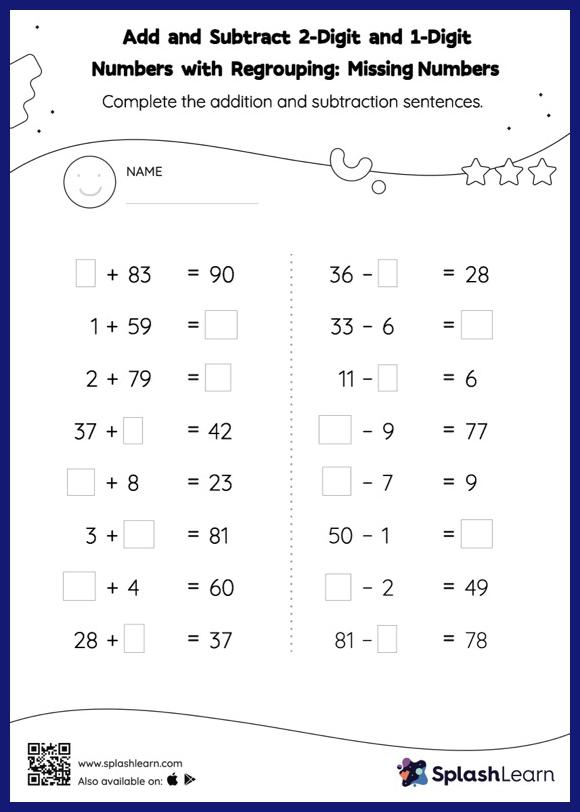# Add and Subtract 2-Digit and 1-Digit Numbers with Regrouping: Missing Numbers Worksheet

Home > Add and Subtract 2-Digit and 1-Digit Numbers with Regrouping: Missing NumbersThis worksheet consists of a set of questions on addition and subtraction where students are required to add and subtract 2-digit and 1-digit numbers with regrouping. In this worksheet, students must find the missing number using the relationship between addition and subtraction. To solve add and subtract 2-digit and 1-digit numbers with regrouping worksheet, they must also regroup the numbers. In each problem, the numbers are laid out in the horizontal format. Students should try to use different strategies involving composing and decomposing numbers to solve these problems. This will help them develop flexibility and fluency.